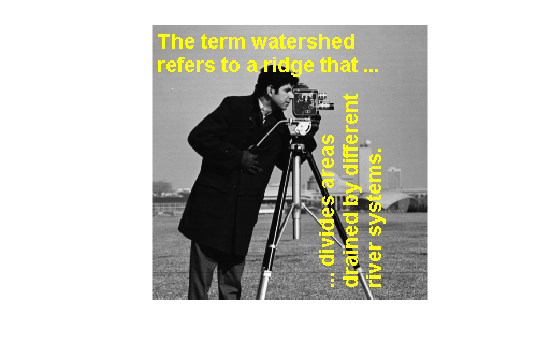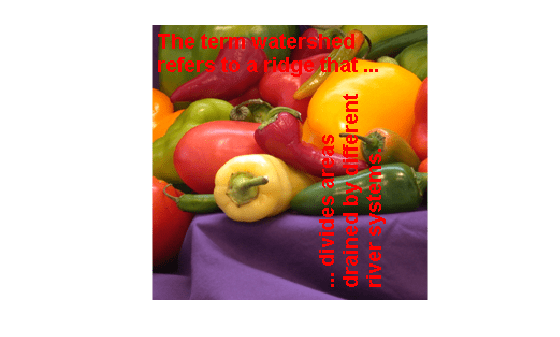# imoverlay

Burn binary mask into 2-D image

## Syntax

``B = imoverlay(A,BW)``
``B = imoverlay(A,BW,color)``

## Description

example

````B = imoverlay(A,BW)` fills the grayscale or RGB image `A` with a solid color where the input binary mask, `BW`, is `true`.```
````B = imoverlay(A,BW,color)` specifies the color that `imoverlay` uses to fill the image.```

## Examples

collapse all

Read a grayscale image into the workspace.

`A = imread('cameraman.tif');`

Read a binary image into the workspace.

`BW = imread('text.png');`

Burn the binary image into the grayscale image, specifying the color to be used for the binary mask.

`B = imoverlay(A,BW,'yellow');`

Display the result.

`imshow(B)`Read an RGB image into the workspace.

`RGB = imread('peppers.png');`

Read a binary image into the workspace.

`BW = imread('text.png');`

Crop the RGB image to make it the same size as the binary mask.

`RGB_cropped = imcrop(RGB,[64,128,255,255]);`

Burn the binary image into the cropped RGB image, choosing the color to be used.

`B = imoverlay(RGB_cropped,BW,'red');`

Display the result.

```figure imshow(B)```## Input Arguments

collapse all

Input image, specified as a 2-D grayscale image or 2-D RGB image.

Data Types: `single` | `double` | `int16` | `uint8` | `uint16` | `logical`

Mask image, specified 2-D binary matrix or 2-D numeric matrix of the same size as the first two dimensions of image `A`. For numeric input, any nonzero pixels are considered to be `1` (`true`).

Data Types: `single` | `double` | `int8` | `int16` | `int32` | `uint8` | `uint16` | `uint32` | `logical`

Color used for the overlay, specified as an RGB triplet, a color name, or a short color name.

You can specify any color using an RGB triplet. An RGB triplet is a 3-element row vector whose elements specify the intensities of the red, green, and blue components of the color. The intensities must be in the range [0, 1].

You can specify some common colors by name as a string scalar or character vector. This table lists the named color options and the equivalent RGB triplets.

Color NameShort NameRGB TripletAppearance
`"red"``"r"``[1 0 0]``"green"``"g"``[0 1 0]``"blue"``"b"``[0 0 1]``"cyan"` `"c"``[0 1 1]``"magenta"``"m"``[1 0 1]``"yellow"``"y"``[1 1 0]``"black"``"k"``[0 0 0]``"white"``"w"``[1 1 1]`Here are the RGB triplets for the default colors that MATLAB® uses in many types of plots.

RGB TripletAppearance
`[0 0.4470 0.7410]``[0.8500 0.3250 0.0980]``[0.9290 0.6940 0.1250]``[0.4940 0.1840 0.5560]``[0.4660 0.6740 0.1880]``[0.3010 0.7450 0.9330]``[0.6350 0.0780 0.1840]`Example: `'r'`

Example: `'green'`

Example: ```[0 0.4470 0.7410]```

## Output Arguments

collapse all

Output image, returned as a 2-D RGB image.

Data Types: `uint8`

## Version History

Introduced in R2016a